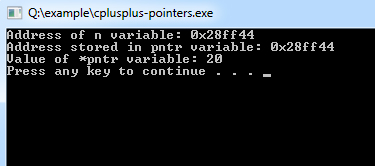## C++ Programming Tutorial Index

The pointer variable in C++ holds the address of a memory location. This tutorial will guide you on how to use the pointer in C++.

For a C++ program, computer memory is like a succession of memory cells, each holding a one-byte size and having a unique address. Dealing with these memory addresses in programming is done by pointers. Pointers are powerful programming tools that can make things easier, help increase program efficiency, and allow programmers to handle large amounts of data. Pointers can allocate memory dynamically, where programmers don't have to worry about how much memory they will need to assign for each task, which cannot be done/performed without the concept of a pointer. Hence pointer is considered the most distinct and exciting feature of the C++ language, which adds power and flexibility to the language.

## Pointer Definition in C++

Syntax:

``type *variable_name;``

Example:

``````int  *height;
char  *age;``````

## Benefits of using Pointers in C++

• Pointers save the memory.
• Pointers reduce the length and complexity of a program.
• Pointers allow the passing of arrays and strings to function more efficiently.
• Pointers make it possible to return more than one value from the function.
• Pointers increase the processing speed.

## How to use Pointers in C++

Example:

``````#include <iostream>
using namespace std;

int main ()
{
int  n = 20, *ptr;  /* actual and pointer variable declaration */

ptr = &n;  /* store address of n in pointer variable*/

cout << "Address of n variable: " << &n << endl;

/* print address stored in pointer variable */
cout << "Address stored in pntr variable: " << ptr << endl;

/* print access the value using the pointer */
cout << "Value of *pntr variable: " << *ptr << endl;

system("pause");
return 0;
}``````

Program Output: